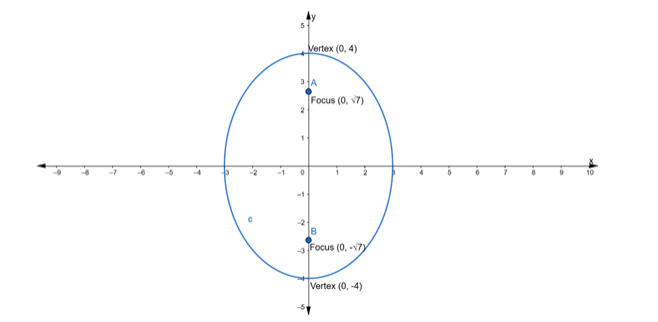# Find the equation of the ellipse whose vertices are the (0, ±4) and foci`
Question:

Find the equation of the ellipse whose vertices are the (0, ±4) and foci at $(0, \pm \sqrt{7})$

Solution:Given: Vertices $=(0, \pm 4) \ldots$ (i)

The vertices are of the form $=(0, \pm a) \ldots$ (ii)

Hence, the major axis is along $y$-axis

$\therefore$ From eq. (i) and (ii), we get

$a=4$

$\Rightarrow a^{2}=16$

and We know that, if the major axis is along y – axis then the equation of Ellipse is of the form of

$\frac{x^{2}}{b^{2}}+\frac{y^{2}}{a^{2}}=1$

Also, given coordinate of foci $=(0, \pm \sqrt{7}) \ldots$ (iii)

We know that

Coordinates of foci $=(0, \pm \mathrm{c}) \ldots$ (iv)

$\therefore$ From eq. (iii) and (iv), we get

$c=\sqrt{7}$

We know that,

$c^{2}=a^{2}-b^{2}$

$\Rightarrow(\sqrt{7})^{2}=(4)^{2}-b^{2}$

$\Rightarrow 7=16-b^{2}$

$\Rightarrow b^{2}=16-7$

$\Rightarrow b^{2}=9$

Substituting the value of $a^{2}$ and $b^{2}$ in the equation of an ellipse, we get

$\frac{x^{2}}{b^{2}}+\frac{y^{2}}{a^{2}}=1$

$\Rightarrow \frac{x^{2}}{9}+\frac{y^{2}}{16}=1$